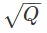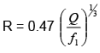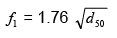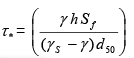Courses

# Geomorphology of Rivers (Part - 3) Civil Engineering (CE) Notes | EduRev

## Civil Engineering (CE) : Geomorphology of Rivers (Part - 3) Civil Engineering (CE) Notes | EduRev

The document Geomorphology of Rivers (Part - 3) Civil Engineering (CE) Notes | EduRev is a part of the Civil Engineering (CE) Course Water Resources Engineering.
All you need of Civil Engineering (CE) at this link: Civil Engineering (CE)

Hydraulic geometry of alluvial rivers

Width, depth, average velocity, average longitudinal bed slope of a natural river depends on many factors like discharge and sediment variation throughout the year and over the years, type of bed material and their variation in the river bed, type of bank material. There have been numerous attempts to find some relations between all the variables, which may be grouped as under (ASCE, 1998):

1. Regime theory and power law approach
2. External hypothesis approach
3. Tractive force methods.

The first of the three methods is traditionally the oldest and some of the initial contributors like Kennedy (1885), Lindley (1919), and Lacey (1929) based their hypotheses on research carried out on small rivers and artificial channels in India. Other noted contributors were Simons and Albertson (1963) and Blench (1969). The latter also based his study on the data gather from Indian rivers. Of the various formulas proposed so far by different researchers, the one by Lacey is quite popular in India though it has its own limitations as pointed out by Lane.

In fact, the data that was analyzed by him pertained to stable artificial channels of north India which were flowing through sandy materials and where carrying relatively small amount of bed material load. The following equations, which were originally presented by Lacey in FPS units, are being given here in terms of SI units

P = 4.75(9)(10)

S = 0.0003 f15/3 / Q1/ 6         (11)

U = 0.516 f1 5/3 / Q1/ 6  = 10.8 R2/3 S1/2                          (12)(13)

In the above expressions, the variables stand for

• P: Wetted Perimeter (m)
• R: Hydraulic Radius (m)
• S: Channel Slope
• U: Average Velocity (m/s)
• Q: Discharge (m3/s)
• f1: Silt factor, a term used to define the fineness or coarseness of the  channel bed material.
• d50: Median size of bed material in mm

In India, hydraulic engineers have used these equations often for rivers, small and large. For wide rivers, the equations for R in terms of discharge q (discharge per unit width) obtained by combining the first two equations is used in the following form:

R = 1.35 ((q2/f1)1/3

A critical analysis of the formulae proposed by Lacey shows that sediment load is not included as one of the independent variables in the equation along with Q and d. Secondly, it has been pointed out by other researchers, that since the formulae of Lacey was based on data from channels of north India where the base is alluvial and banks are cohesive, the proposed equations are applicable largely to channels and canals with sandy bed and cohesive bars. Further the regime equations, as the equation proposed by Lacey are generally called, correspond to a given bank full discharge.

In the recent years the work by Julien and Wargadalam (1995) has attempted to refine the regime approach within a framework based on the governing principles of open channel flow. After studying data from 835 non-cohesive alluvial rivers and canals they arrived at the following relationship

h = 0.2 Q 0.33 d50 0.17 Sf -0.17                 (15)

W = 1.33  Q 0.44 d50 -0.11 Sf -0.22             (16)

U = 3.76 Q0.22 d50-0.05 Sf0.39                   (17)

Sf = 0.121 Q 0.33 d50 -0.83 Sf0.33                       (18)

These are the simplified formulae of the more detailed ones and have been showed to agree quite well measured data. The variable sin the above equations are defined as follows

• h: Flow Depth (m)
• W: Water surface width (m)
• U: Average Velocity (m/s)
• Sf: Friction Slope
• d50: Median slope of bed material (m)

The simplified equations proposed by Julian and Wargadalam are suitable whenτ*= 0.047, when Manning’s equation is generally applicable to describe flow in the river. Higher segment transport implies higher velocity and slope and reduced width and depth. It may be recalled that Shield’s parameter τ* is defined as:(19)

Two other approaches that have been proposed for describing the hydraulic geometry of an alluvial river are based on height following theories:

a) Extremal hypothesis

b) Tractive force approaches

These have been more commonly applied in the recent years. But the method proposed by Lane in threshold channel theory has also been used often to design canals in alluvium.

Offer running on EduRev: Apply code STAYHOME200 to get INR 200 off on our premium plan EduRev Infinity!

## Water Resources Engineering

158 docs|16 tests

,

,

,

,

,

,

,

,

,

,

,

,

,

,

,

,

,

,

,

,

,

;